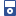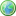Accessibility options:

# The Argand Diagram and Polar form resources

Show me all resources applicable to

### 3GP Mobile Phone (2)The Argand Diagram
This mobile phone video explains how complex numbers can be represented pictorially using an Argand Diagram. Sigma resource Unit 8. This resource is released under a Creative Commons license Attribution-Non-Commercial-No Derivative Works and the copyright is held by mathcentre.The modulus and argument of a complex number
This mobile phone video explains how to calculate the modulus and argument of a complex number. Sigma resource Unit 9. This resource is released under a Creative Commons license Attribution-Non-Commercial-No Derivative Works and the copyright is held by mathcentre.

### Guides (1)Just the Maths (A.J.Hobson)
"Just the Maths" authored by the late Tony Hobson, former Senior Lecturer in Mathematics of the School of Mathematical and Information Sciences at Coventry University, is a collection of separate mathematics units, in chronological topic-order, intended for foundation level and first year degree level in higher education where mathematics is a service discipline e.g. engineering.

### iPOD Video (2)The Argand Diagram
This mp4 video explains how complex numbers can be represented pictorially using an Argand Diagram. Sigma resource Unit 8. This resource is released under a Creative Commons license Attribution-Non-Commercial-No Derivative Works and the copyright is held by mathcentre.The modulus and argument of a complex number
This mp4 video explains how to calculate the modulus and argument of a complex number. Sigma resource Unit 9. This resource is released under a Creative Commons license Attribution-Non-Commercial-No Derivative Works and the copyright is held by mathcentre.

### Quick Reference (7)Multiplication and division in polar form
This leaflet explains how complex numbers in polar form can be multiplied and divided. In polar form, these operations are particularly simple to carry out.(Engineering Maths First Aid Kit 7.6)The Argand diagram
This leaflet explains how an Argand diagram is used to provide a pictorial representation of a complex number. (Engineering Maths First Aid Kit 7.3)The Argand Diagram
This leaflet explains how complex numbers can be represented pictorially using an Argand Diagram. There are accompanying videos. Sigma resource Unit 8.The form r(cos(t) + j sin(t))
This leaflet explains how a complex number can be written in the form z=r(cos(t) + j sin(t)). (Engineering Maths First Aid Kit 7.5)The modulus and argument of a complex number
This leaflet explains how to calculate the modulus and argument of a complex number. There are accompanying videos. Sigma resource Unit 9.The polar form
This leaflet explains the polar form of a complex number. It defines the modulus and argument of a complex number. (Engineering Maths First Aid Kit 7.4)The polar form of a complex number
This leaflet explains what is meant by the polar form of a complex number. There are accompanying videos. Sigma resource Unit 10.

### Test Yourself (5)Basic complex numbers - Numbas
6 questions on complex numbers, multiplication, inverse, division and modulus. Finding the distance between complex numbers. Numbas resources have been made available under a Creative Commons licence by Bill Foster and Christian Perfect, School of Mathematics & Statistics at Newcastle University.Complex Numbers Test 01 (DEWIS)
Seven questions on complex numbers. Testing modulus, multiplication, division, Argand diagram, polar form, De Moivre's theorem. DEWIS resources have been made available under a Creative Commons licence by Rhys Gwynllyw & Karen Henderson, University of the West of England, Bristol.Maths EG
Computer-aided assessment of maths, stats and numeracy from GCSE to undergraduate level 2. These resources have been made available under a Creative Common licence by Martin Greenhow and Abdulrahman Kamavi, Brunel University.Modulus and argument - Numbas
Find the modulus and argument of complex numbers. One question. Numbas resources have been made available under a Creative Commons licence by Bill Foster and Christian Perfect, School of Mathematics & Statistics at Newcastle University.Polar Form and De Moivre's Theorem - Numbas
3 questions. Finding modulus and argument of complex numbers. Use De Moivre's Theorem to find powers of complex numbers. Numbas resources have been made available under a Creative Commons licence by the School of Mathematics & Statistics at Newcastle University.

### Video (3)The Argand Diagram
This video explains how complex numbers can be represented pictorially using an Argand Diagram. Sigma resource Unit 8. This resource is released under a Creative Commons license Attribution-Non-Commercial-No Derivative Works and the copyright is held by mathcentre.The modulus and argument of a complex number
This video explains how to calculate the modulus and argument of a complex number. Sigma resource Unit 9. This resource is released under a Creative Commons license Attribution-Non-Commercial-No Derivative Works and the copyright is held by mathcentre.The polar form of a complex number
This video explains what is meant by the polar form of a complex number. Sigma resource Unit 10. This resource is released under a Creative Commons license Attribution-Non-Commercial-No Derivative Works and the copyright is held by mathcentre.

Website design by Pink Mayhem, Leicester